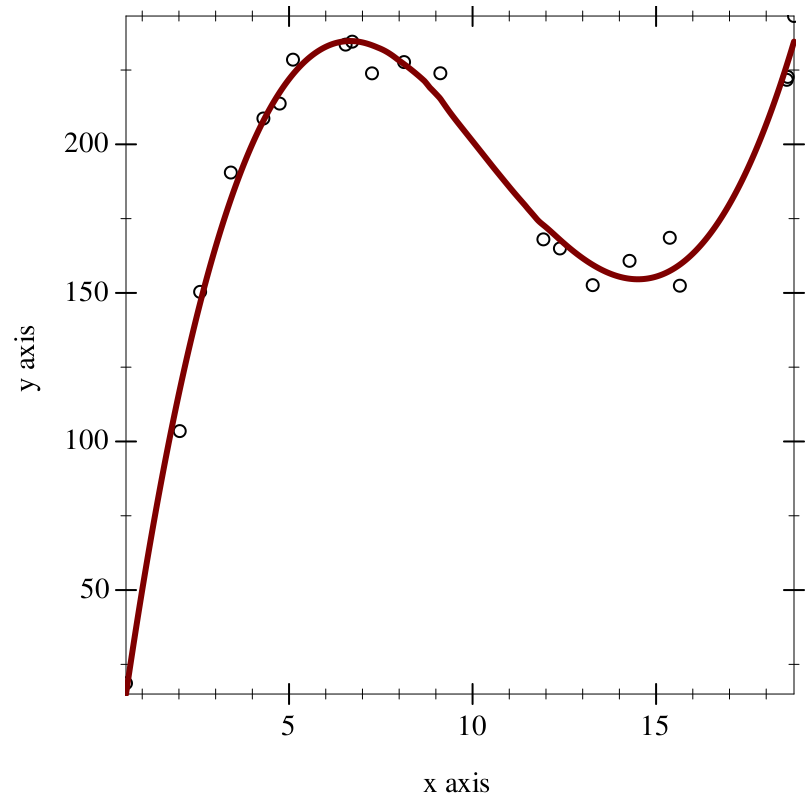8.3

## Loess: local polynomial regression

 (require loess) package: loess

Loess is a library for calculating lines of best fit using local polynomial regression, which is a non-parametric regression method that calculates various individual regressions for each point. It is useful for when you have data that does not follow a clearly discernable pattern, or when you just want a smooth line for a data visualization.

 procedure(loess-fit xs ys [#:span span #:degree degree]) → (-> Real Real) xs : (Vectorof Real) ys : (Vectorof Real) span : Real = 0.75 degree : Positive-Integer = 1
Creates a line of best fit from the input data. The output is a function which cannot be cleanly represented as an equation, designed to be passed to something such as plot’s function renderer.

xs and ys are assumed to be vectors of the same length, with each observation being at the same index in each vector.

The optional keyword argument #:span dictates the proportion of the data to factor into each local regression. For example, if the span is 0.1, the local regression will use the (* 0.1 (vector-length xs)) nearest neighbors to the input point. Generally, increasing #:span increases the influence of bias in the resulting model, and decreasing it increases the influence of variance. This number is assumed to be between (/ (add1 degree) (vector-length xs)) and 1 (as otherwise there are not enough points to create a fit).

The optional keyword argument #:degree dictates the degree of each local regression, and not the degree of the complete regression.

If the result is suboptimal or jittery, changing #:span and #:degree is generally the key. If the line appears too flat, generally you want to reduce #:span, and if it appears too chaotic, generally you want to increase #:span.

For example, a noisy signal that should look like a sine wave:
 > (define pure-xs : (Vectorof Real) (vector-sort (ann (build-vector 200 (λ _ (* (random) 4.0 pi))) (Vectorof Real)) <))
 > (define noisy-ys : (Vectorof Real) (vector-map (λ ([x : Real]) (+ (* 2 (sin x)) (* 1.5 (random)))) pure-xs))
 > (plot (list (points (vector-map (inst vector Real) pure-xs noisy-ys)) (function (loess-fit pure-xs noisy-ys #:span 0.2) #:width 3)))

- : plot-pictAnother example, with a higher-degree fit:
 > (define xx : (Vectorof Real) (vector 0.5578196 2.0217271 2.5773252 3.4140288 4.3014084 4.7448394 5.1073781 6.5411662 6.7216176 7.2600583 8.1335874 9.1224379 11.9296663 12.3797674 13.2728619 14.2767453 15.3731026 15.6476637 18.5605355 18.5866354 18.7572812))
 > (define yy : (Vectorof Real) (vector 18.63654 103.49646 150.35391 190.51031 208.70115 213.71135 228.49353 233.55387 234.55054 223.89225 227.68339 223.91982 168.01999 164.9575 152.61107 160.78742 168.55567 152.42658 221.70702 222.6904 243.18828))
 > (plot (list (points (vector-map (inst vector Real) xx yy)) (function (loess-fit xx yy #:degree 3) #:width 3)))

- : plot-pictNote while this appears like a 3rd-degree polynomial fit, it is actually not a 3rd-degree polynomial.